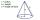# Square prism

A square prism has a base with a length of 23 centimeters, what is the area in square centimeters of the base of the prism?

Result

A =  529 cm2

#### Solution:Leave us a comment of example and its solution (i.e. if it is still somewhat unclear...):Be the first to comment!## Next similar examples:

1. The diagramThe diagram is a cone of radius 8cm and height 10cm. The diameter of the base is. ..
2. Street numbersLada came to aunt. On the way he noticed that the houses on the left side of the street have odd numbers on the right side and even numbers. The street where he lives aunt, there are 5 houses with an even number, which contains at least one digit number 6.
3. Find xSolve: if 2(x-1)=14, then x= (solve an equation with one unknown)
4. WithdrawalIf I withdrew 2/5 of my total savings and spent 7/10 of that amount. What fraction do I have in left in my savings?
5. Negative in equation2x + 3 + 7x = – 24, what is the value of x?
6. DecideThe rectangle is divided into seven fields. On each box is to write just one of the numbers 1, 2 and 3. Mirek argue that it can be done so that the sum of the two numbers written next to each other was always different. Zuzana (Susan) instead argue that.
7. Pizza 4Marcus ate half pizza on monday night. He than ate one third of the remaining pizza on Tuesday. Which of the following expressions show how much pizza marcus ate in total?
8. Unknown number 11That number increased by three equals three times itself?
9. RoundingThe following numbers round to the thousandth:
10. TeacherTeacher Rem bought 360 pieces of cupcakes for the outreach program of their school. 5/9 of the cupcakes were chocolate flavor and 1/4 wete pandan flavor and the rest were vanilla flavor. How much more chocolate flavor cupcakes than vanilla flavor?
11. NormThree workers planted 3555 seedlings of tomatoes in one dey. First worked at the standard norm, the second planted 120 seedlings more and the third 135 seedlings more than the first worker. How many seedlings were standard norm?
12. AlleyAlley measured a meters. At the beginning and end are planted poplar. How many we must plant poplars to get the distance between the poplars 15 meters?
13. Write 2Write 791 thousandths as fraction in expanded form.
14. Simple equationSolve the following simple equation: 2. (4x + 3) = 2-5. (1-x)
15. Round it0.728 round to units, tenths, hundredths.
16. DisjointHow many elements have union and intersection of two disjoint sets when the first have 1 and secodn 8 elements.
17. Equation 29Solve next equation: 2 ( 2x + 3 ) = 8 ( 1 - x) -5 ( x -2 )